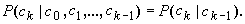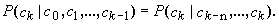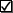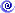Introduction

The Viterbi Algorithm made its first appearance in the coding literature in a paper written by A. J. Viterbi  in 1967. Since then, due to its easyness in implementation, it has been applied to many different areas related to decoding problems.

In general, the decoding problem is as follows: Given a model and a sequence of observations, what is the most likely state sequence in the model that produces the observations? Suppose we have an observation sequence Z=(z0,z1,...,zn) and we want to check if that sequence is a correct one. The idea is to find the sequence C=(c0,c1,...,cn) which is the closest to sequence Z. Each ci in C, for i=0,1,...,n, can take any value of the states given in the model. Let M be the number of states in the model. Fig. 1 summarizes the decoding problem.Fig. 1 The decoding problem.

Now, the question is, how can we know which sequence C is the closest to sequence Z? Different types of techniques have been developed, the most attractives ones are based on compound decision theory, where probability plays an important role. One of those techniques is the Viterbi algorithm, which gives an optimal solution to the decoding problem if the underlying process is assumed to be a Markov process. In the next section we will give details about compound decision theory, since it is crucial to better understand how the Viterbi algorithm works. We now define a Markov process.

Markov process

A Markov process is characterized as follows. The state ck at time k is one of a finite number in the range {1,..,M}. Under the assumption that the process runs only from time 0 to time n and that the initial and final states are known, the state sequence is then represented by a finite vector C=(c0,...,cn).

Let P(ck | c0,c1 ,...,ck-1) denote the probability (chance of occurrence) of the state ck at time k conditioned on all states up to time k-1. The process is a first-order Markov, in the sense that the probability of being in state ck at time k, given all states up to time k-1 depends only on the previous state, i.e. ck-1 at time k-1:For an nth-order Markov process,In general, for the Viterbi algorithm, the underlying process is assumed to be a Markov process with the following characteristics:finite-state, this means that the number M is finite.discrete-time, this means that going from one state to other takes the same unit of time.observed in memoryless noise, this means that the sequence of observations depends probabilistically only on the previous sequence transitions.

In this tutorial we explain how the Viterbi algorithm works, and specifically, expose its use in the area of Text Recognition. Forney  and Neuhoff  are considered among the first researchers to propose the VA to the text recognition problem.

Shinghal et. al.  conducted an experimental investigation of text-recognition by four methods. The methods of classification are: without contextual information, Raviv's recursive Bayes algorithm , the modified Viterbi algorithm , and a proposed heuristic approximation to Raviv's algorithm. In general, the problem of text recognition has been thoroughly analyzed with the final goal of extracting contextual information contained in words that a machine might be able to make use of. This relevant information can be categorized in six classes of word features as mentioned in :Graphological features are those that characterize the shape of a word; examples of this are:the height-to-width ratio of the word (gestalt graphological feature)printing stylePhonological features are those that characterize the sound of words, as in the acoustic similarity of rhyme.Semantic features consists of markers indicating the meaning of words, i.e. taxonomic categories.Pragmatic features are related to the information about how the user uses a word.Statistical features are present in words in the sense of frequency, the more we see a word the more easily we recognized it.Syntactic features of words are markers for parts of speech.Contextual information can also be expressed in terms of statistical or syntatic rules concerning the relations among letters in a word. For example: the frequencies of occurrence of letters, letter pairs (bigrams), and letter triplets(trigrams).

The use of contextual information in Pattern Recognition to solve the text recognition problem has focused more in this latter category, as we will see in detail later on.